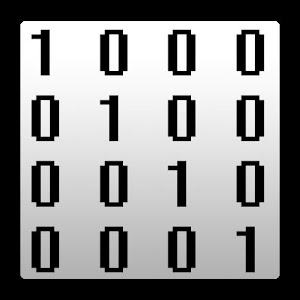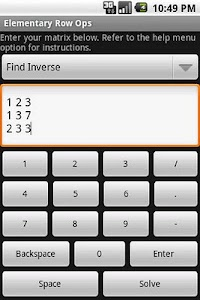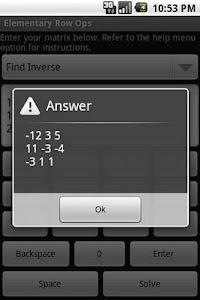# Elementary Row Operations – Solve a system of equations or find the inverse or determinant of a matrix.Solve a system of equations or find the inverse or determinant of a matrix.Can help in a class like Linear Algebra. Solve a system of equations or find the inverse or determinant of a matrix. Use spaces between numbers in a row and a new line between rows. Numbers can be decimals or fractions; 0.75, .75, 3/4, and 1.5/2 are valid.